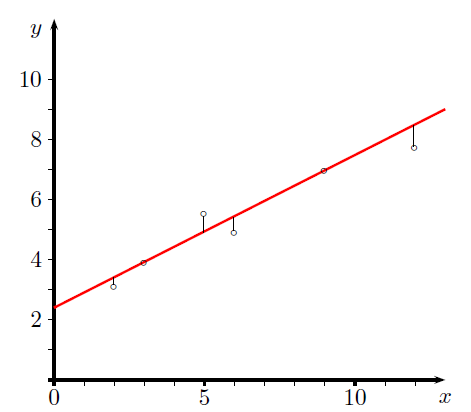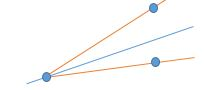# Why can’t we use absolute values of Errors? (Least Squares Method)- Curve Fitting1. If we look at the above image and the data points we notice that some of the errors will be positive and some will be negative. The problem is that when we add positive and negative values, they tend to cancel each other out.
2. Squaring emphasizes larger differences.
3. But most importantly it’s because whenever we have a formula that we want to maximize or minimize, we can use Calculus to find that maximum or minimum. We use a process called differentiation.

Ask any Calculus professor (or student who has taken Calculus) how they minimize a formula using Calculus. They should quickly be able to tell you something along the lines of, “Oh, you just take the first derivative and set it equal to zero.” Now show them the formula we want to minimize. In fact, point out the absolute value bars on the formula and watch as the look of fear or dread overcomes them. That’s because absolute values are difficult to work with in mathematics (especially Calculus). In fact, for what we want to do, we cannot use our formula with absolute values. In mathematical terms, we would say this is because the use of absolute values results in discontinuous derivatives that cannot be treated analytically.

We simply choose not to use absolute values because of the difficulties we have in working with them mathematically.

The criterion of making the magnitudes (absolute values) minimum is awkward because the absolute value function has no derivative at the origin, and it is also felt to give undue importance to large errors.

Also, assume that two of the points are at the same x-value (which is not an abnormal situation, as frequently experiments are duplicated). The best line will obviously pass through the average of the duplicated tests. However, any line that falls between the red lines shown will have the same sum of the magnitude of the vertical distances. We wish an unambiguous result so we cannot use this as the basis for our work.[wpedon id="7041" align="center"]